# Digital Media Primer YueLing Wong Copyright c2013 by

• Slides: 46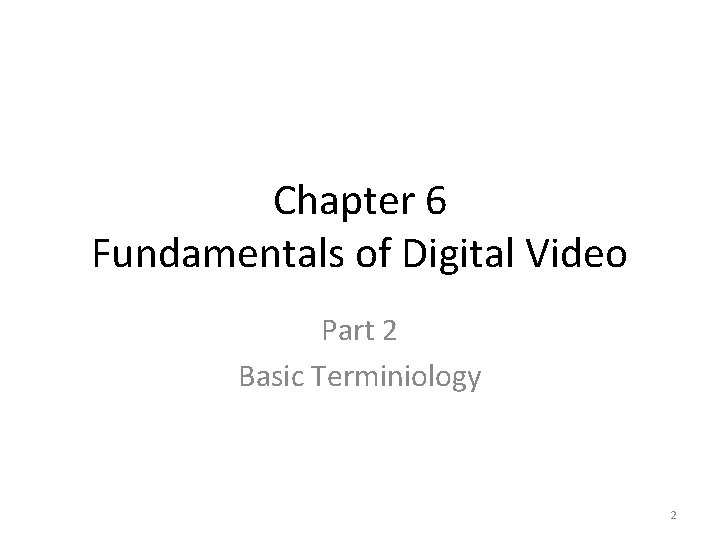Chapter 6 Fundamentals of Digital Video Part 2 Basic Terminiology 2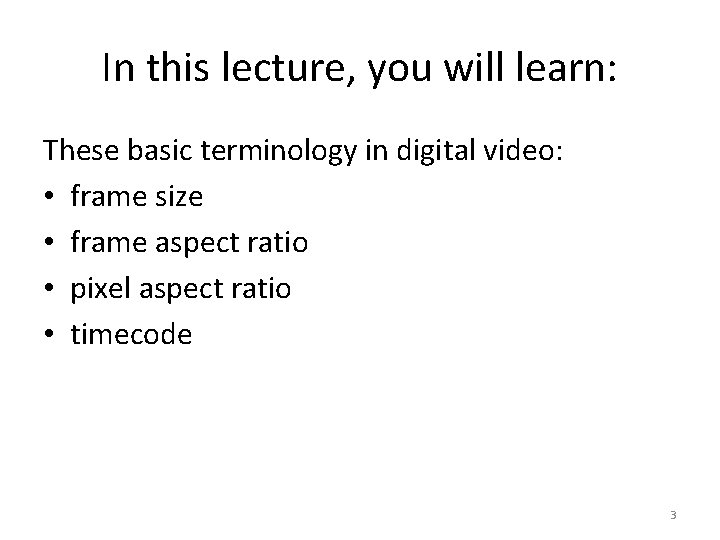In this lecture, you will learn: These basic terminology in digital video: • frame size • frame aspect ratio • pixel aspect ratio • timecode 3Sampling and Quantization of Motion • Temporal: – sampling rate: • • how frequent you take a snapshot of the motion frame rate higher sampling rate: higher frame rate more frames for the same duration larger file size 4Sampling and Quantization of Motion • Each snapshot: a frame – an image – digitized based on the same concepts of sampling and quantization of images 5Frame Size • Resolution of the frame image • Measured in pixel dimensions • No ppi setting: Unlike digital images, there is no pixel per inch (ppi) setting for video because video is not intended for print but for on screen display. 6Frame Size Examples NTSC PAL standard definition high definition HDV format standard definition Frame size 720 x 480 pixels 1280 x 720 pixels 1440 x 1080 pixels 720 x 576 pixels 7Frame Aspect Ratio • the ratio of a frame's viewing width to height • NOT equivalent to ratio of the frame’s pixel width to height. 8Frame Aspect Ratio Examples 4: 3 Example: • Standard definition NTSC standard format 16: 9 Examples: • Standard definition NTSC wide-screen format • High definition digital video • High definition TV 9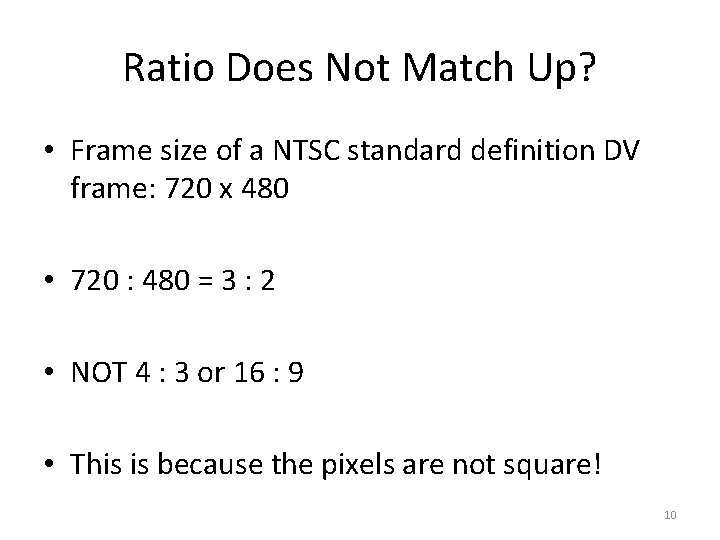Ratio Does Not Match Up? • Frame size of a NTSC standard definition DV frame: 720 x 480 • 720 : 480 = 3 : 2 • NOT 4 : 3 or 16 : 9 • This is because the pixels are not square! 10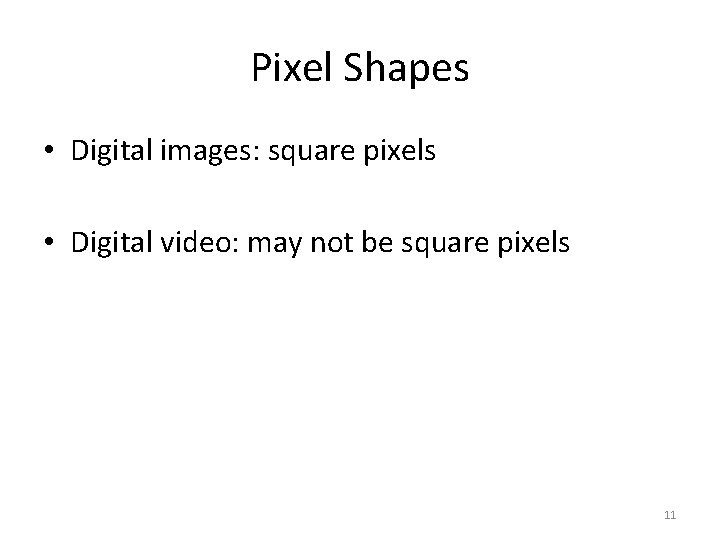Pixel Shapes • Digital images: square pixels • Digital video: may not be square pixels 11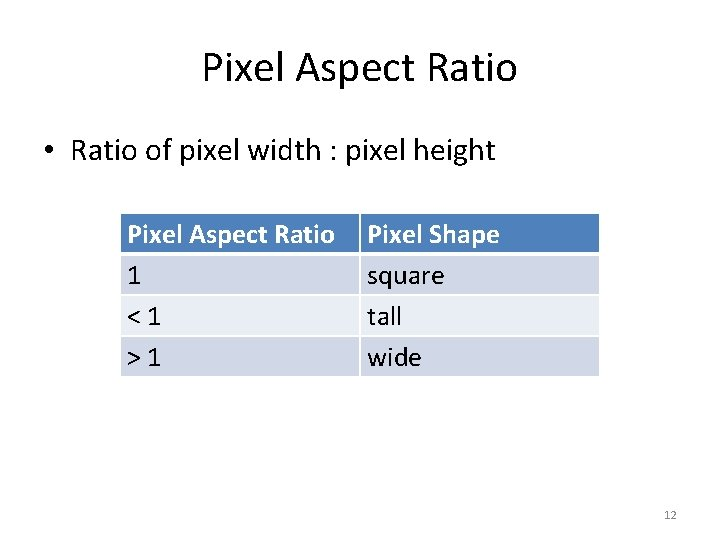Pixel Aspect Ratio • Ratio of pixel width : pixel height Pixel Aspect Ratio 1 <1 >1 Pixel Shape square tall wide 12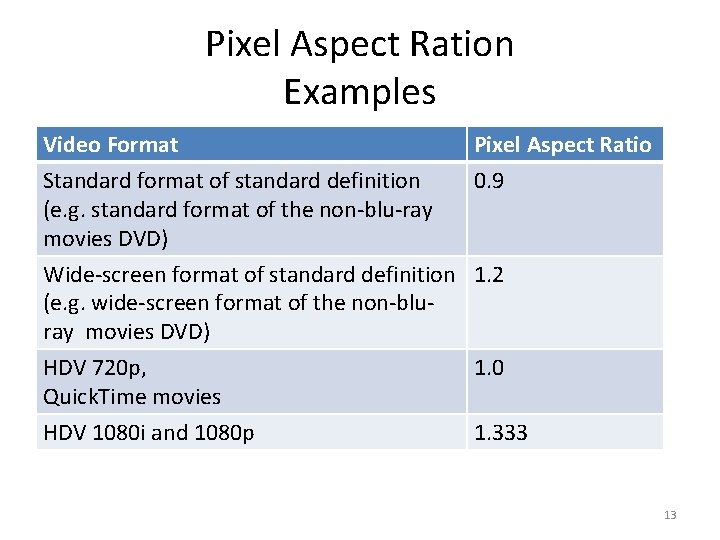Pixel Aspect Ration Examples Video Format Pixel Aspect Ratio Standard format of standard definition 0. 9 (e. g. standard format of the non-blu-ray movies DVD) Wide-screen format of standard definition 1. 2 (e. g. wide-screen format of the non-bluray movies DVD) HDV 720 p, Quick. Time movies 1. 0 HDV 1080 i and 1080 p 1. 333 13Let's see if the ratios match up 14Standard Format Standard Definition • Pixel aspect ratio = 0. 9 • Frame size = 720 480 • Frame aspect ratio = 720 0. 9 : 480 = 648 : 480 4: 3 15Wide-screen Format Standard Definition • Pixel aspect ratio = 1. 2 • Frame size = 720 480 • Frame aspect ratio = 720 1. 2 : 480 = 864: 480 16 : 9 16HDV 720 p • Pixel aspect ratio = 1. 0 • Frame size = 1280 720 • Frame aspect ratio = 1280 1. 0 : 720 = 1280 : 720 = 16 : 9 17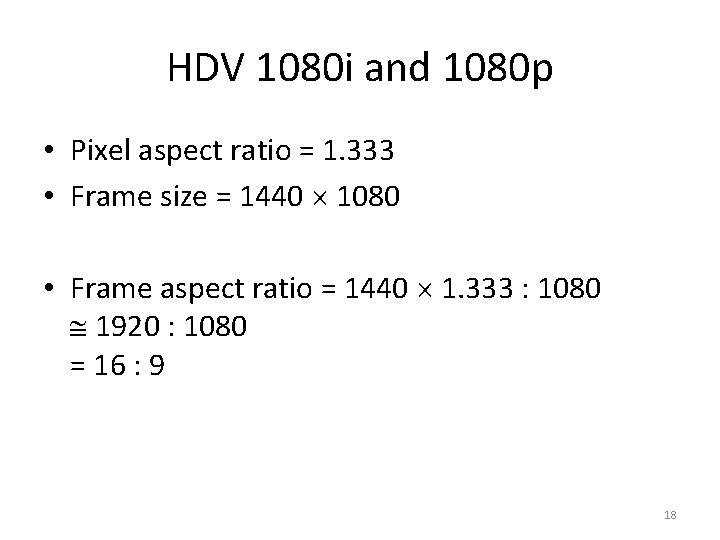HDV 1080 i and 1080 p • Pixel aspect ratio = 1. 333 • Frame size = 1440 1080 • Frame aspect ratio = 1440 1. 333 : 1080 1920 : 1080 = 16 : 9 18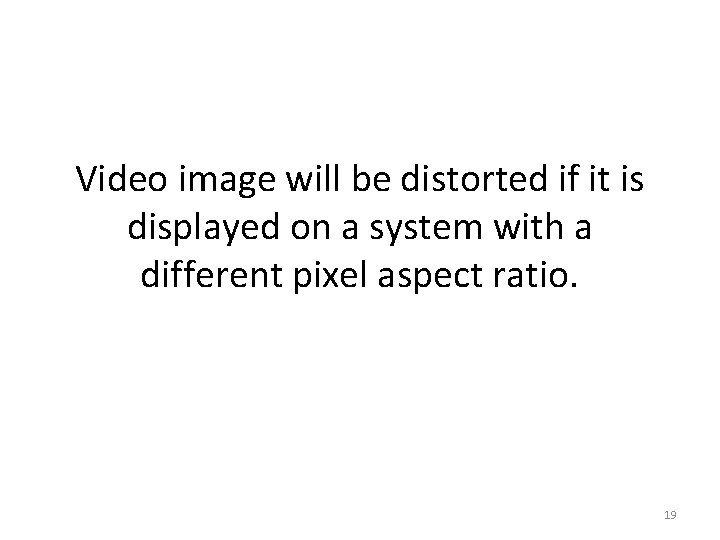Video image will be distorted if it is displayed on a system with a different pixel aspect ratio. 19For a video of pixel aspect ratio of 1 Displayed correctly on a system of pixel aspect ratio of 1 Displayed incorrectly on a system of pixel aspect ratio of 0. 9. The image looks stretched vertically. 20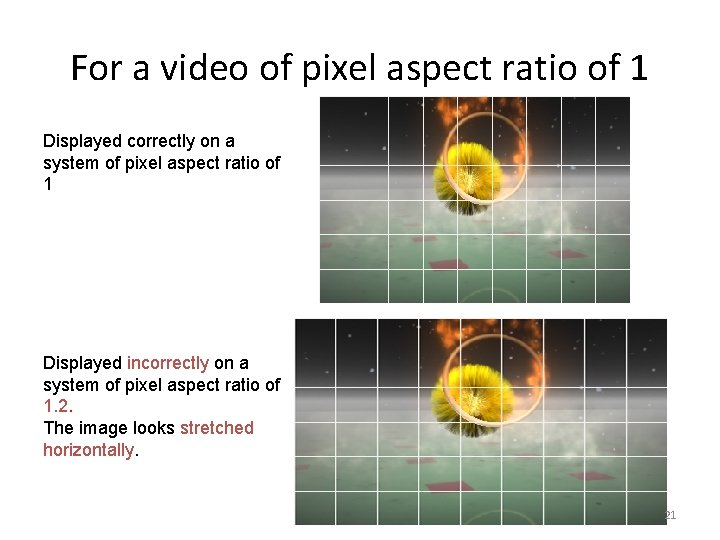For a video of pixel aspect ratio of 1 Displayed correctly on a system of pixel aspect ratio of 1 Displayed incorrectly on a system of pixel aspect ratio of 1. 2. The image looks stretched horizontally. 21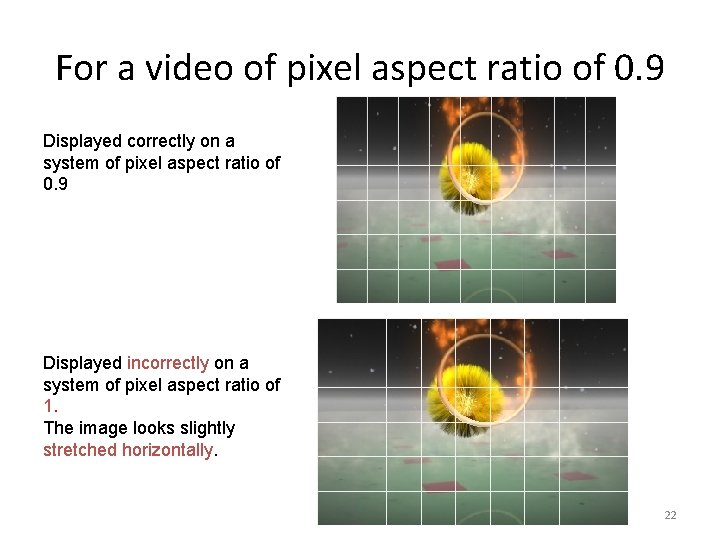For a video of pixel aspect ratio of 0. 9 Displayed correctly on a system of pixel aspect ratio of 0. 9 Displayed incorrectly on a system of pixel aspect ratio of 1. The image looks slightly stretched horizontally. 22For a video of pixel aspect ratio of 0. 9 Displayed correctly on a system of pixel aspect ratio of 0. 9 Displayed incorrectly on a system of pixel aspect ratio of 1. 2. The image looks stretched horizontally. 23For a video of pixel aspect ratio of 1. 2 Displayed correctly on a system of pixel aspect ratio of 1. 2 Displayed incorrectly on a system of pixel aspect ratio of 0. 9. The image looks stretched vertically. 24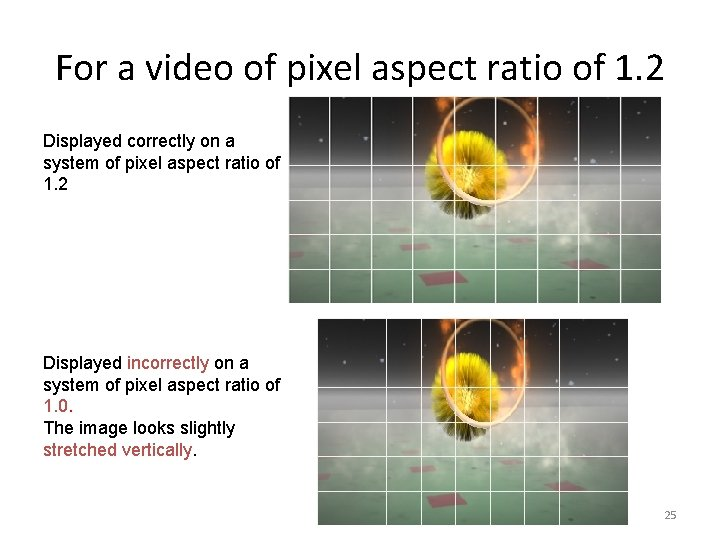For a video of pixel aspect ratio of 1. 2 Displayed correctly on a system of pixel aspect ratio of 1. 2 Displayed incorrectly on a system of pixel aspect ratio of 1. 0. The image looks slightly stretched vertically. 25Also see the interactive tutorial: PIXEL ASPECT RATIO AN INTERACTIVE DEMO SHOWS YOU THE EFFECT OF AN INCORRECT PIXEL ASPECT RATIO ON AN IMAGE. 26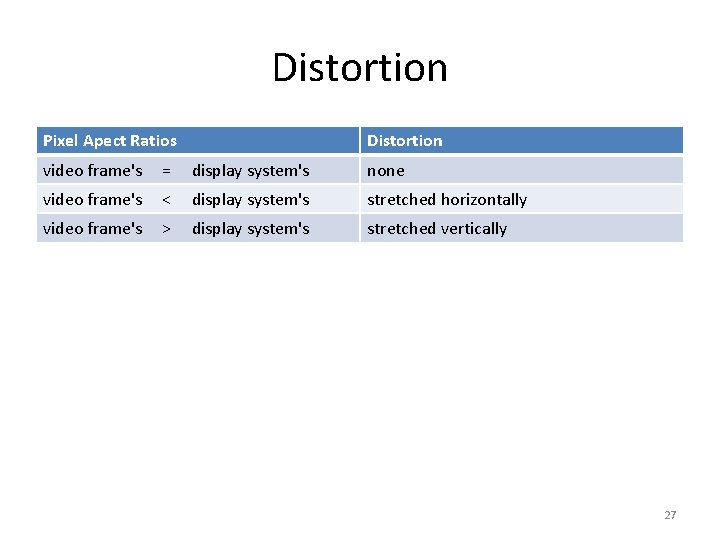Distortion Pixel Apect Ratios Distortion video frame's = display system's none video frame's < display system's stretched horizontally video frame's > display system's stretched vertically 27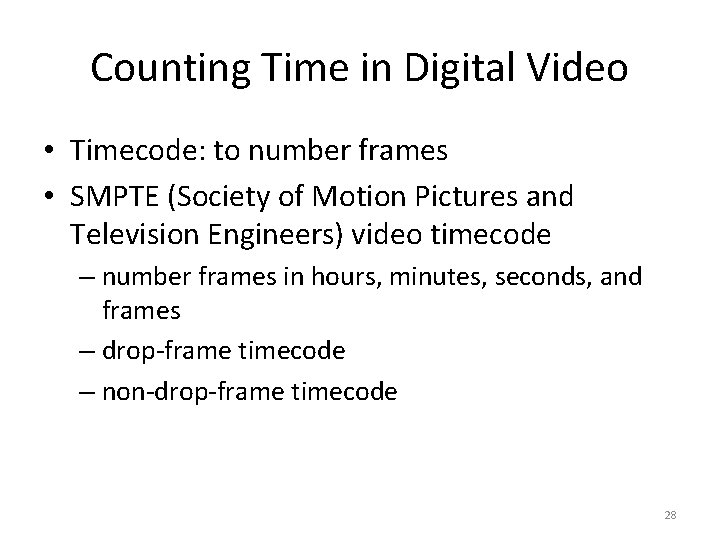Counting Time in Digital Video • Timecode: to number frames • SMPTE (Society of Motion Pictures and Television Engineers) video timecode – number frames in hours, minutes, seconds, and frames – drop-frame timecode – non-drop-frame timecode 28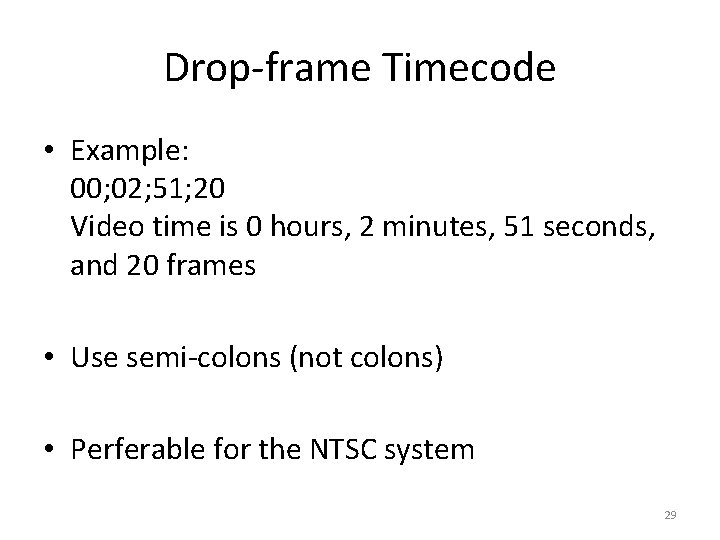Drop-frame Timecode • Example: 00; 02; 51; 20 Video time is 0 hours, 2 minutes, 51 seconds, and 20 frames • Use semi-colons (not colons) • Perferable for the NTSC system 29Drop-frame Timecode • Does NOT mean dropping or removing frames from the video • NTSC video is 29. 97 fps, not exactly 30 fps • Drop-frame timecode renumbers frames to make the timecode more accurately represent the video time based on 29. 97 fps 30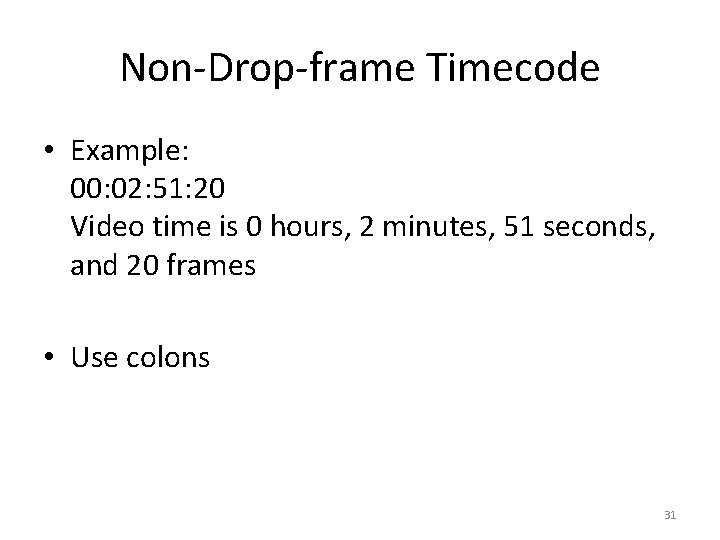Non-Drop-frame Timecode • Example: 00: 02: 51: 20 Video time is 0 hours, 2 minutes, 51 seconds, and 20 frames • Use colons 31Review Questions Note to instructor: Depending on your preference, you may want to go over the review questions at the end of this lecture as an instant review or at the beginning of next lecture to refresh students' memory of this lecture. 32Review Question True/False: There is no sampling and quantization involved in capturing motion in digital video. 33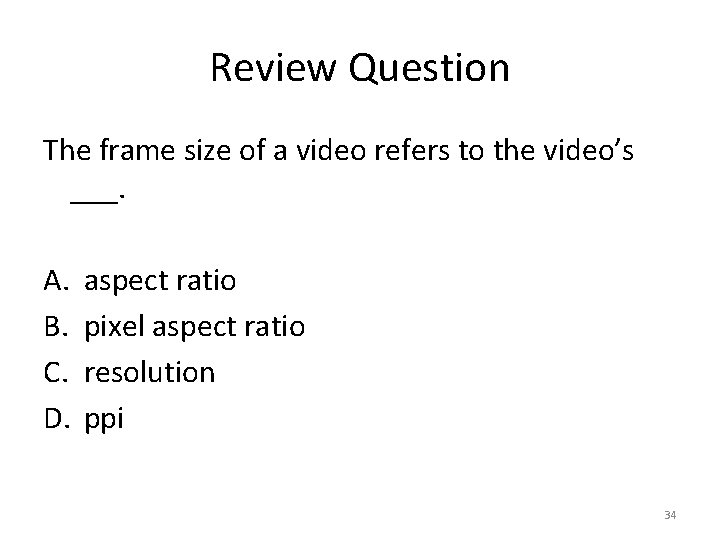Review Question The frame size of a video refers to the video’s ___. A. B. C. D. aspect ratio pixel aspect ratio resolution ppi 34Review Question True/False: The pixel per inch (ppi) is an important attribute for video resolution and should be set correctly when working with digital video in video-editing programs. 35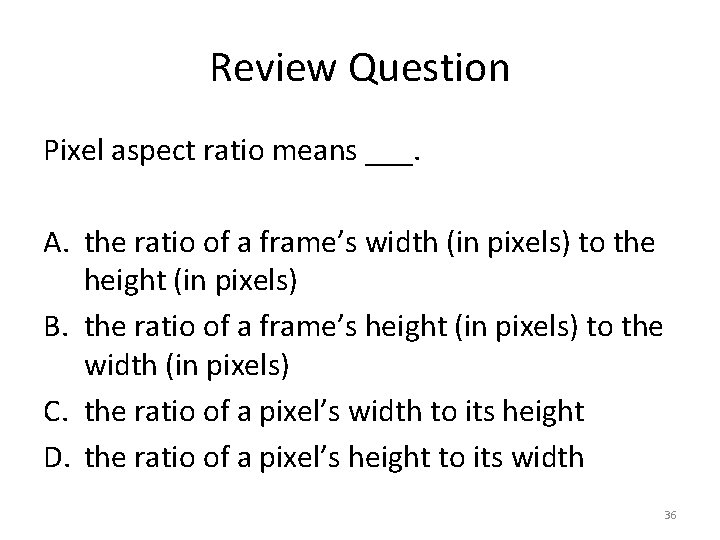Review Question Pixel aspect ratio means ___. A. the ratio of a frame’s width (in pixels) to the height (in pixels) B. the ratio of a frame’s height (in pixels) to the width (in pixels) C. the ratio of a pixel’s width to its height D. the ratio of a pixel’s height to its width 36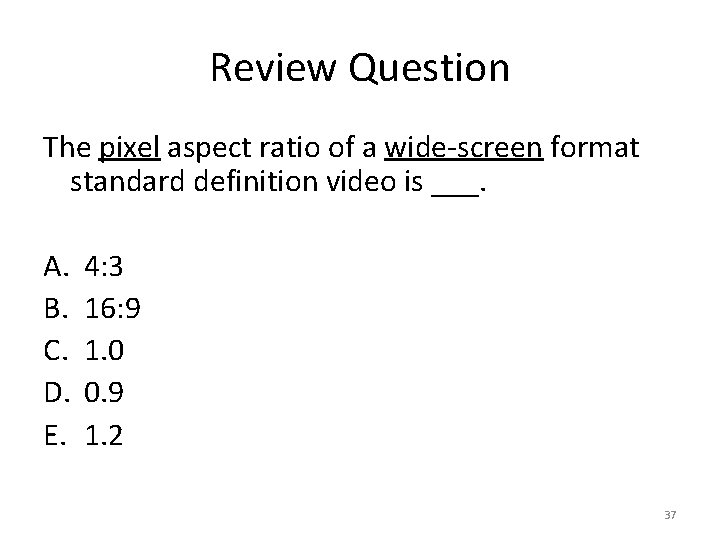Review Question The pixel aspect ratio of a wide-screen format standard definition video is ___. A. B. C. D. E. 4: 3 16: 9 1. 0 0. 9 1. 2 37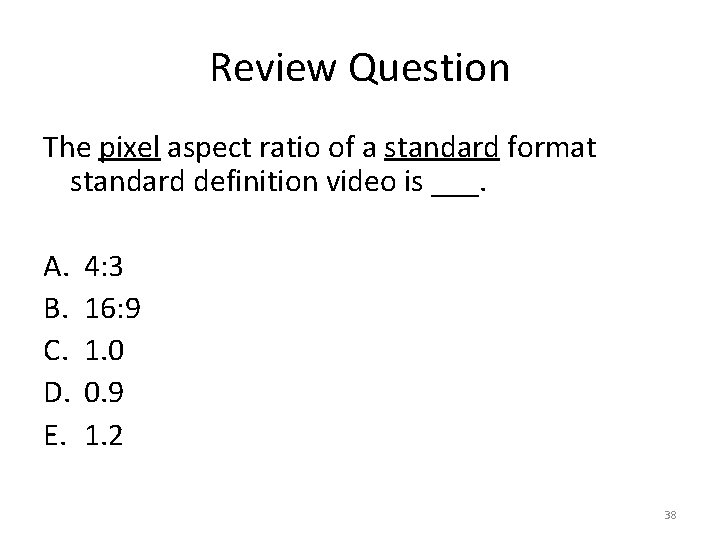Review Question The pixel aspect ratio of a standard format standard definition video is ___. A. B. C. D. E. 4: 3 16: 9 1. 0 0. 9 1. 2 38Review Question The frame aspect ratio of a wide-screen format standard definition video is ___. A. B. C. D. E. 4: 3 16: 9 1. 0 0. 9 1. 2 39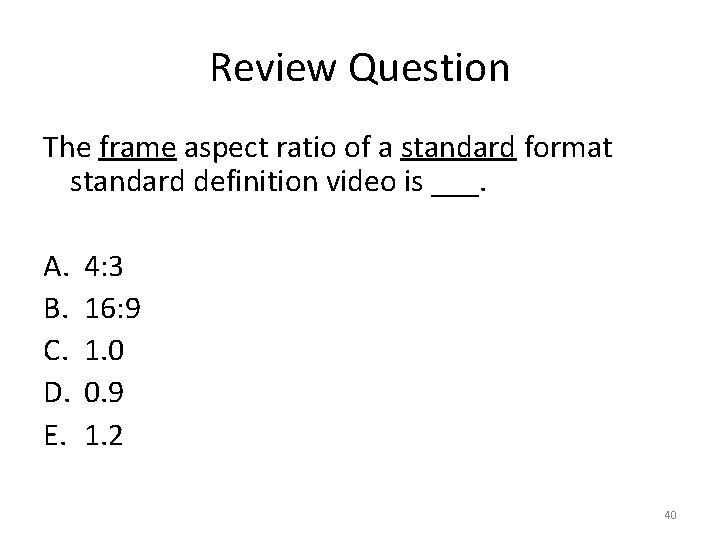Review Question The frame aspect ratio of a standard format standard definition video is ___. A. B. C. D. E. 4: 3 16: 9 1. 0 0. 9 1. 2 40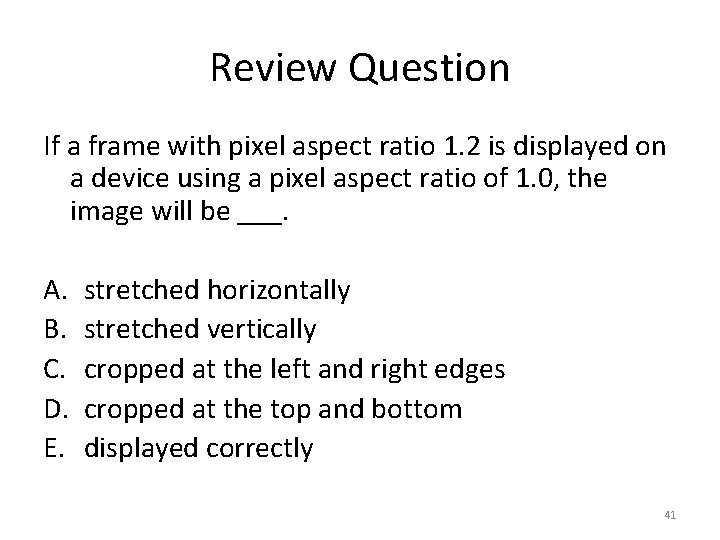Review Question If a frame with pixel aspect ratio 1. 2 is displayed on a device using a pixel aspect ratio of 1. 0, the image will be ___. A. B. C. D. E. stretched horizontally stretched vertically cropped at the left and right edges cropped at the top and bottom displayed correctly 41Review Question If a frame with pixel aspect ratio of 1 is displayed on a device using a pixel aspect ratio of 1. 2, the image will look like ___. A. B. 42Review Question True/False: The timecode representing the 35 th frame is either 00: 00: 35 or 00; 00; 35. 43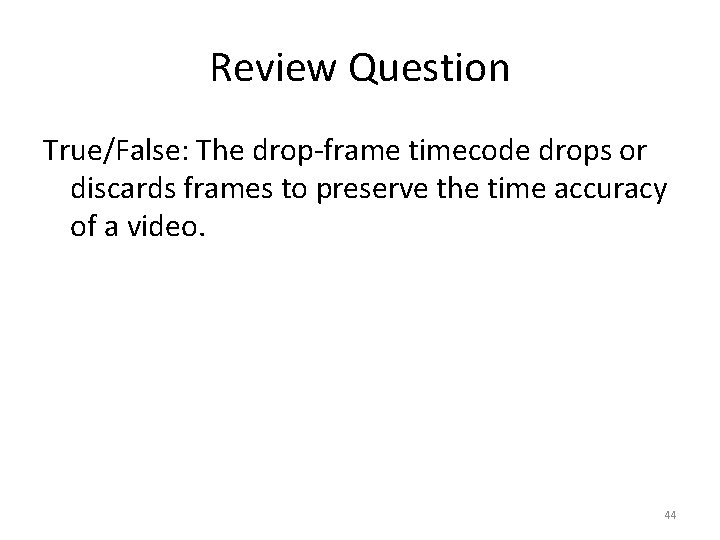Review Question True/False: The drop-frame timecode drops or discards frames to preserve the time accuracy of a video. 44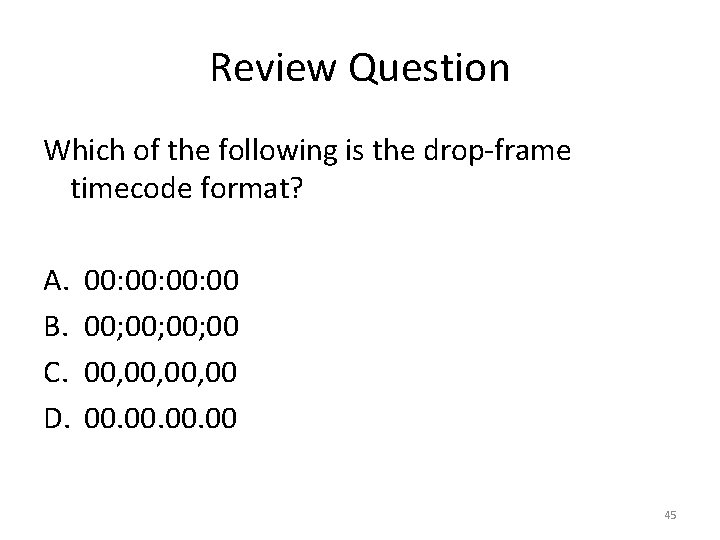Review Question Which of the following is the drop-frame timecode format? A. B. C. D. 00: 00: 00 00; 00; 00 00, 00, 00 00. 00. 00 45Review Question Which timecode format is preferable for the NTSC system? A. drop-frame B. non-drop-frame 46